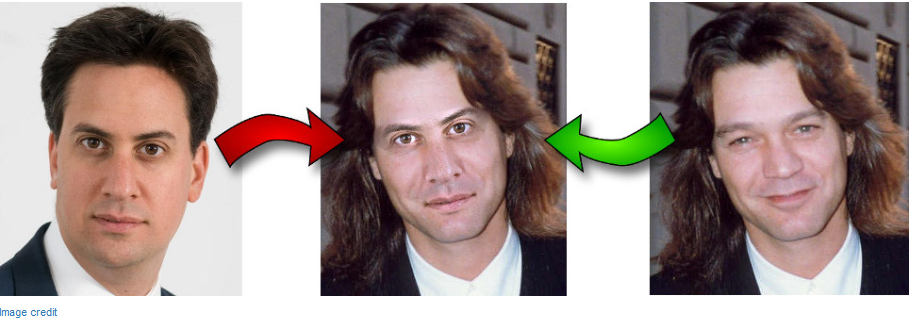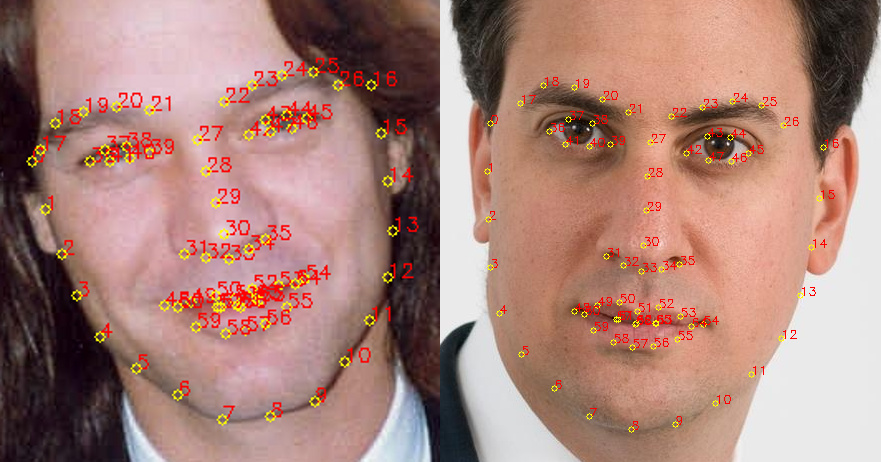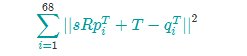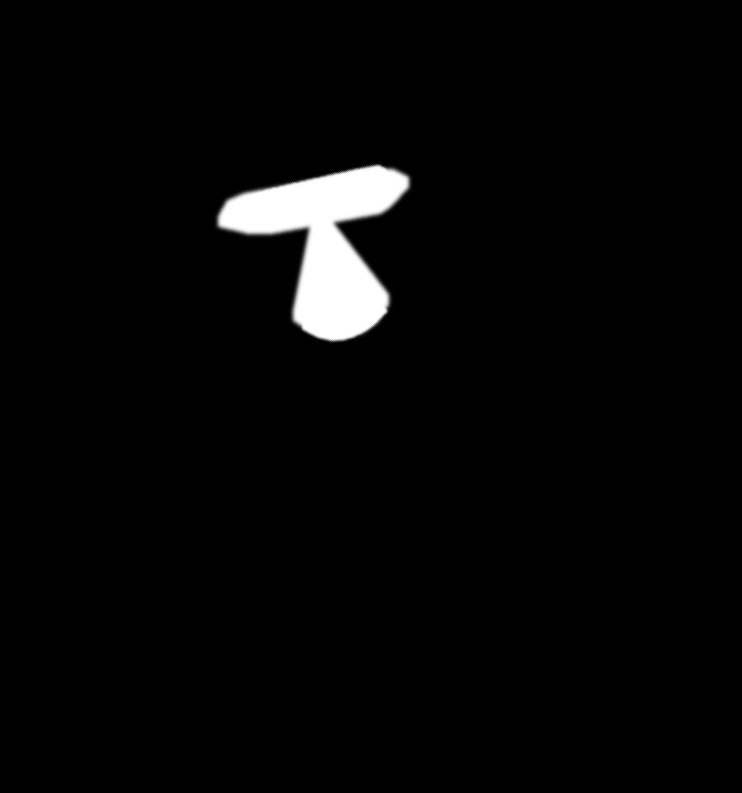## 教你用200行Python代码“换脸” 原

r
ranvane## 介绍

• 检测面部标记。
• 旋转、缩放和转换第二张图像，使之与第一张图像相适应。
• 调整第二张图像的色彩平衡，使之与第一个相匹配。
• 把第二张图像的特性混合在第一张图像中。

1．使用dlib提取面部标记``PREDICTOR_PATH = "/home/matt/dlib-18.16/shape_predictor_68_face_landmarks.dat" detector = dlib.get_frontal_face_detector() predictor = dlib.shape_predictor(PREDICTOR_PATH) def get_landmarks(im): rects = detector(im, 1) if len(rects) > 1: raise TooManyFaces if len(rects) == 0: raise NoFaces return numpy.matrix([[p.x, p.y] for p in predictor(im, rects).parts()])``

get_landmarks()函数将一个图像转化成numpy数组，并返回一个68 x2元素矩阵，输入图像的每个特征点对应每行的一个x，y坐标。

2．用普氏分析(Procrustes analysis)调整脸部R是个2 x2正交矩阵，s是标量，T是二维向量，pi和qi是上面标记矩阵的行。

``````def transformation_from_points(points1, points2): points1 = points1.astype(numpy.float64) points2 = points2.astype(numpy.float64) c1 = numpy.mean(points1, axis=0) c2 = numpy.mean(points2, axis=0) points1 -= c1
points2 -= c2

s1 = numpy.std(points1) s2 = numpy.std(points2) points1 /= s1
points2 /= s2

U, S, Vt = numpy.linalg.svd(points1.T * points2) R = (U * Vt).T return numpy.vstack([numpy.hstack(((s2 / s1) * R, c2.T - (s2 / s1) * R * c1.T)), numpy.matrix([0., 0., 1.])])``````

``def warp_im(im, M, dshape): output_im = numpy.zeros(dshape, dtype=im.dtype) cv2.warpAffine(im, M[:2], (dshape, dshape), dst=output_im, borderMode=cv2.BORDER_TRANSPARENT, flags=cv2.WARP_INVERSE_MAP) return output_im``3．校正第二张图像的颜色``COLOUR_CORRECT_BLUR_FRAC = 0.6 LEFT_EYE_POINTS = list(range(42, 48)) RIGHT_EYE_POINTS = list(range(36, 42)) def correct_colours(im1, im2, landmarks1): blur_amount = COLOUR_CORRECT_BLUR_FRAC * numpy.linalg.norm( numpy.mean(landmarks1[LEFT_EYE_POINTS], axis=0) - numpy.mean(landmarks1[RIGHT_EYE_POINTS], axis=0)) blur_amount = int(blur_amount) if blur_amount % 2 == 0: blur_amount += 1 im1_blur = cv2.GaussianBlur(im1, (blur_amount, blur_amount), 0) im2_blur = cv2.GaussianBlur(im2, (blur_amount, blur_amount), 0) # Avoid divide-by-zero errors. im2_blur += 128 * (im2_blur <= 1.0) return (im2.astype(numpy.float64) * im1_blur.astype(numpy.float64) / im2_blur.astype(numpy.float64))``4．把第二张图像的特性混合在第一张图像中``````LEFT_EYE_POINTS = list(range(42, 48)) RIGHT_EYE_POINTS = list(range(36, 42)) LEFT_BROW_POINTS = list(range(22, 27)) RIGHT_BROW_POINTS = list(range(17, 22)) NOSE_POINTS = list(range(27, 35)) MOUTH_POINTS = list(range(48, 61)) OVERLAY_POINTS = [ LEFT_EYE_POINTS + RIGHT_EYE_POINTS + LEFT_BROW_POINTS + RIGHT_BROW_POINTS, NOSE_POINTS + MOUTH_POINTS, ] FEATHER_AMOUNT = 11 def draw_convex_hull(im, points, color): points = cv2.convexHull(points) cv2.fillConvexPoly(im, points, color=color) def get_face_mask(im, landmarks): im = numpy.zeros(im.shape[:2], dtype=numpy.float64) for group in OVERLAY_POINTS: draw_convex_hull(im, landmarks[group], color=1) im = numpy.array([im, im, im]).transpose((1, 2, 0)) im = (cv2.GaussianBlur(im, (FEATHER_AMOUNT, FEATHER_AMOUNT), 0) > 0) * 1.0 im = cv2.GaussianBlur(im, (FEATHER_AMOUNT, FEATHER_AMOUNT), 0) return im

• 这样一个遮罩同时为这两个图像生成，使用与步骤2中相同的转换，可以使图像2的遮罩转化为图像1的坐标空间。

• 之后，通过一个element-wise最大值，这两个遮罩结合成一个。结合这两个遮罩是为了确保图像1被掩盖，而显现出图像2的特性。

``output_im = im1 * (1.0 - combined_mask) + warped_corrected_im2 * combined_mask``r

### ranvane

AI科技大本营
01/29
0
0
20行Python代码给微信头像戴帽子

AI科技大本营
01/16
0
0

在我们开始今天的内容之前，请允许我们再强调一遍：所有我们教授的Deepfake技术仅用于正义的学习，并且禁止被用于其他任何邪恶的用途！ 　　如果提到2018年初最“黑科技”的AI应用是什么...

2018/04/17
0
0
【子豪兄】十行Python代码实现人脸识别

Bilibili视频教程：十行Python代码实现人脸识别 本章内容 使用基于Haar特征的Cascade级联分类器进行人脸识别（听起来好高大上，但其实原理很简单） 用人脸识别同样的道理，扩展到人眼识别上 ...

05/20
0
0

eminemning
2018/05/21
0
0

Redis集群搭建

jxlgzwh
5分钟前
1
0
avro

hexiaoming123
6分钟前
1
0
QML TextInput的字体超出控件范围

shzwork
8分钟前
1
0
《Java 8 in Action》Chapter 10：用Optional取代null

1965年，英国一位名为Tony Hoare的计算机科学家在设计ALGOL W语言时提出了null引用的想法。ALGOL W是第一批在堆上分配记录的类型语言之一。Hoare选择null引用这种方式，“只是因为这种方法实...

HelloDeveloper
9分钟前
1
0

11分钟前
1
0